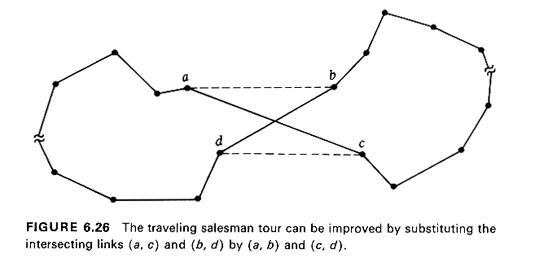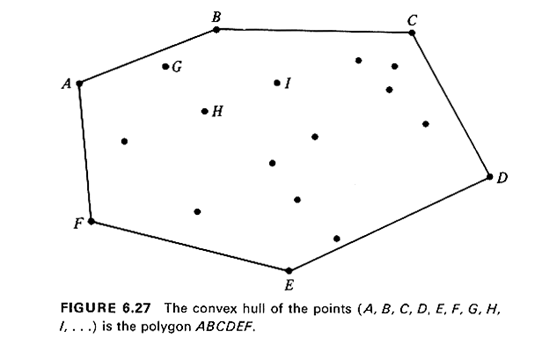### 6.4.7 Euclidean TSP

When travel is Euclidean in TSP1, we have the Euclidean (or "geometrical") TSP. It has been shown that, theoretically, the Euclidean TSP is equally hard with the general TSP, in the same sense that TSP1 is just as hard as the general TSP [PAPA 77].

Nonetheless, the Euclidean TSP is probably the easiest version of TSP for finding good approximate solutions, either manually or with the aid of the computer. For one, since the Euclidean TSP is just a special case of TSP1, all heuristics that have been designed for TSP1 (such as Algorithm 6.6) can also be used for the Euclidean TSP. Second, the following two properties hold in the case of the Euclidean TSP.

Property 1: The optimum traveling salesman tour does not intersect itself.

Property 2: Let m of the n points in the Euclidean TSP define the convex hull (see below) of the points. Then the order in which these m points appear in the optimum traveling salesman tour must be the same as the order in which these same points appear on the convex hull.

The validity of Property I is obvious: two intersecting links on a tour can always be replaced by two nonintersecting links whose total length is guaranteed to be less due to the triangle inequality (see Figure 6.26).With regard to Property 2 (see Figure 6.27), the convex hull of a set of points in a two-dimensional Euclidean space is defined as the smallest (in terms of area) convex polygon that includes all the points in the set. For the case shown in Figure 6.27, a tour that consists, in part, of the sequence {. . . , A, . . . , C, . . . , B. . . . , D . . .} cannot be optimum according to Property 2, since the points A, B. C, and D do not appear in the tour in the same order as in the convex hull ABCDEF. The validity of Property 2 is a direct consequence of Property 1. (Why ?)

It is obvious that the foregoing two properties reduce enormously the number of candidate tours that must be considered in a geometrical TSP, when the number of points n is large. Because it is much easier for a person than for a computer to detect tour link intersections (as well as violations of Property 2), the two properties are particularly convenient to use when approximate manual solutions to geometrical TSP,s are attempted. Indeed, they provide the conceptual basis for some very intuitive heuristic algorithms for the Euclidean TSP [CROE 58, WIOR 75] and for an excellent "man-machine interactive" algorithm for vehicle routing problems [KROL 72], which will be described briefly later in this chapter.

Finally, we note that for Euclidean TSP,s Property 1 can easily be incorporated in Step 4 of Algorithm 6.6 by modifying the procedure to say: "Check for nodes of G (points) which are visited more than once in the Eulerian tour and for intersecting links on the Eulerian tour and improve.... "